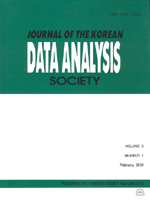상세검색
최근 검색어 전체 삭제
다국어입력
즐겨찾기0KCI등재 학술저널

# Statistical Models for Fog Advisory over Jindo Area

• 등재여부 : KCI등재
• 2010.10
• 2419 - 2429 (11 pages)

This study aims to develop statistical models for the fog advisory over the Jindo area. For this study, visually observed visibility and numerical model outputs are used. All of them are 3-hourly data. As potential predictors, numerical model outputs consist of four kinds of meteorological factors; temperature, depression of dew point temperature, U and V at five layers(surface, 1000hPa, 950hPa, 900hPa and 850hPa) which are generated from the RDAPS in the KMA during three years (from 2006 to 2008). The 3-hour difference data of four factors are added to the set of potential predictors. In this paper, the visibility range for the sea fog advisory is defined by ‘less than 500m’. EGARCH model, ordinal logistic regression model, decision trees and neural networks are applied based on the MOS(model output statistics) method. Their POD(probability of detection) and FAR(false alarm rate) are compared to find the optimal forecast model.

1. Introduction

2. Data and methodology

3. Results

4. Concluding remarks

References